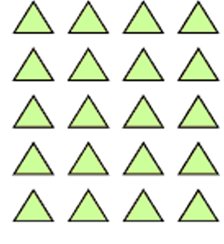Mathematics
Easy

Question

# This array shows 5 × __.## 2    4    5    3Hint:

## The correct answer is: 4

### In the question there is an array using which we have to find the multiplication expression of the given array.The number of columns in the given array= 4The number of rows in the given array= 5So, the multiplication expression of the array will be 5×4.Therefore, the correct option is b, i.e., 4.

For simple multiplication we have to remember the multiplication table of one-digit numbers and for two-digit number we can find it using the multiplication table of one-digit numbers. Here, we have to find the product of one digit number.

### Related Questions to study#### With Turito Foundation.#### Get an Expert Advice From Turito.